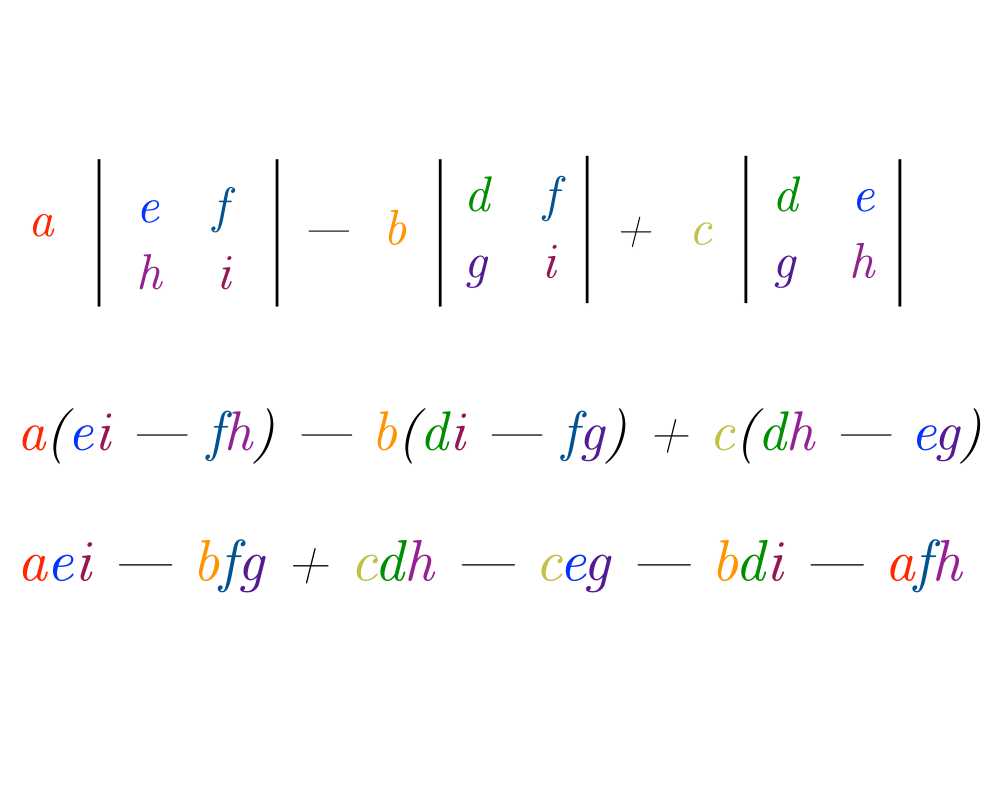# Determinants and PermanentsInputOutput

Input Description: An $$n x n$$ matrix $$M$$.
Problem: What is the determinant $$|M|$$ or the permanent $$Perm(M)$$ for matrix $$m$$?

Excerpt from The Algorithm Design Manual: Determinants of matrices provide a clean and useful abstraction in linear algebra that can used to solve a variety of problems:

• Testing whether a matrix is singular, meaning that the matrix does not have an inverse. A matrix $$M$$ is singular iff $$|M| = 0$$.
• Testing whether a set of $$d$$ points lies on a plane in fewer than $$d$$ dimensions. If so, the system of equations they define is singular, so $$|M| = 0$$.
• Testing whether a point lies to the left or right of a line or plane. This problem reduces to testing whether the sign of a determinant is positive or negative.
• Computing the area or volume of a triangle, tetrahedron, or other simplicial complex. These quantities are a function of the magnitude of the determinant.

A closely related function, called the permanent, arises often in combinatorial problems. For example, the permanent of the adjacency matrix of a graph $$G$$ counts the number of perfect matchings in $$G$$.

Go To Main Page